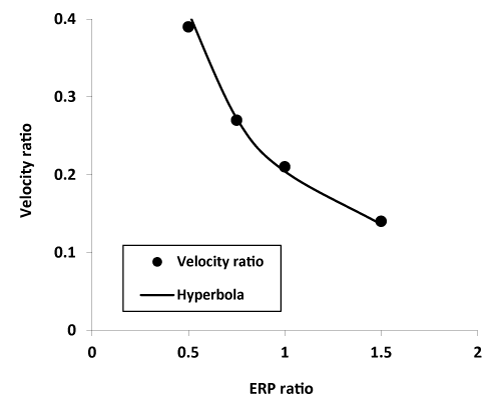Figure 9: Border in two dimensional parameter space between stable physiologic conditions, above the curve, and unstable physiologic conditions, below the curve. Here the parameters refer to conditions in the localized region of abnormal or ischemic tissue, namely the relative reduction in impulse conduction velocity on the vertical axis and relative reduction in refractory period on the horizontal axis. Data points come from numerical experiments. The smooth curve is a hyperbola that represents a constant product of the two variables or 0.21 (y=0.21/x). This curve represents a nearly 80 percent reduction in impulse wavelength in the abnormal region of muscle.